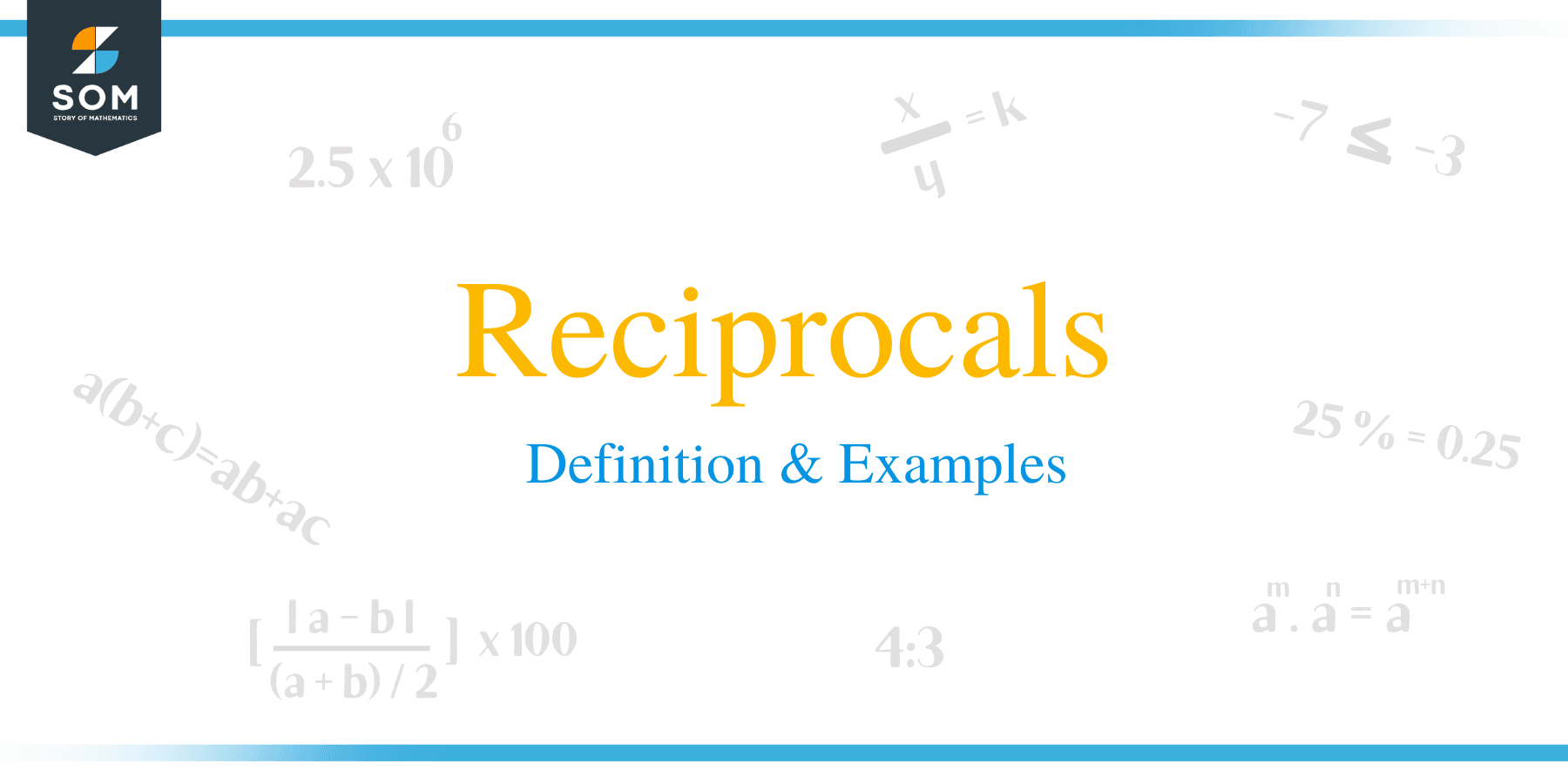# Reciprocals – Definition & Examples

##What is a Reciprocal?

In mathematics, the reciprocal, also known as multiplicative inverse, is the inverse of a number x. denoted as 1/x or x-1. This means that the product of a number x and its reciprocal yields 1.

The inverse of a fraction a/b is denoted as (a/b)-1, which is b/a. This article discusses the steps on how to find the reciprocal of a number, mixed numbers, fractions and decimals.## How to Find Reciprocals?

The reciprocal of a number is simply the number that has been flipped or inverted upside-down. This entails transposing a number such that the numerator and denominator are placed at the bottom and top respectively.

To find the reciprocal of a whole number, just convert it into a fraction in which the original number is the denominator and the numerator is 1.

Example 1

The reciprocal of 2/3 is 3/2.

The product of 2/3 and its reciprocal 3/2 is 1.

2/3 x 3/2 = 1

Example 2

The reciprocal of a whole number 7 is 1/7 because 7 x 1/7 = 1.

### How to Find the Reciprocal of a Mixed Number?

In order to find the reciprocal of a mixed fraction, convert it into improper fraction first and then apply the same rule we learnt above.

Example 3

Find the reciprocal of 4 1/2.

Solution

• Convert a mixed fraction into an improper fraction as calculated below.

4 1/2 = {(4 x 2) + 1}/ 2

= 9/2

• Now flip the numerator and denominator of 9/2.
• Therefore, the solution for the reciprocal of 4 1/2 is 2/9.

### How to Find the Reciprocal of Decimal Numbers?

Like other numbers, decimal numbers too have reciprocals. Calculating the reciprocal of a decimal number can be done in the following ways:

• Convert the decimal into an equivalent fraction, for example, 0.25 = 1/4, and therefore, the reciprocal is 4/1 = 4.
• You can also use a calculate to divide 1 by the fraction. For example, the reciprocal of 0.25 = 1/0.25 = 4It can be noted that dividing 1 by a fraction is the same as multiplying the reciprocal of the number by 1. For example,

5 ÷ 1/4

= 5 x 4/1

= 20

Example 4

Solve the following problems:

a. Find the reciprocal of 5

Solution

5 = 5/1

So, the reciprocal of 3 = 1/5

b. Find the reciprocal of 1/4

Solution

To find the reciprocal of 1/4, invert the numerator and denominator.

The reciprocal of 1/4 = 4

c. Determine the reciprocal of 10/3

Solution

Step 1:

To find the reciprocal of 10/3, flip the numerator and denominator. The reciprocal = 3/10.

Example 5

If 4/7 of a number x is 84. What is the value of x?

Solution
4/7 of a number x = 84
Write the mathematical equation:

(4/7) x = 84

Multiply both sides by the reciprocal of 4/7
Number x = 84 × 7/4

= 21 × 7
= 147
And thus, the number x is 147.

Example 6

A half of the students in a college are boys, 3/5 of these boys take science courses and the rest take humanities. What fraction of the boys take humanities?

Solution

Fraction of boys in the college = 1/2

Fraction of boys who take sciences = 3/5 of 1/2

= 3/5 × 1/2

= 3 × 1/5 × 2

= 3/10

Therefore, 3/10 of the boys take humanities.

Example 7

Pedro has written three-fifth of his 75 paged research work. How many pages are left to complete writing his research?

Solution
Number of pages written = 3/5 of 75
= 3/5 × 7

= 45 pages.
Number of pages left= 75 – 45.
= 30 pages.

Example 8

A herd of cows in a farm produces 99 liters of milk daily. If each cow produces one-third of total milk produced in a day. How many cows are in the farm if 7700 liters of milk is produced weekly.

Solution
A herd of cows produces 99 liters of milk daily.
One cow produces 1/3 of total milk daily = 1/3 of 99
Therefore, one cow produces 11 liters.
Total number of animals in the farm= (7700/7) ÷ 11
= 100 cows

### Practice Questions

1. Which of the following shows the reciprocal of $\dfrac{4}{5}$?

2. Which of the following shows the reciprocal of $6$?

3. Which of the following shows the reciprocal of $3 \dfrac{2}{3}$?

4. Which of the following shows the reciprocal of $12$?

5. Which of the following shows the reciprocal of $\dfrac{1}{5}$?

6. If $3/5$ of a number is $12$. Which of the following shows the correct value of the number?

7. Peter has written three-fifth of his 75 paged research work. How many pages are left to complete writing his research?